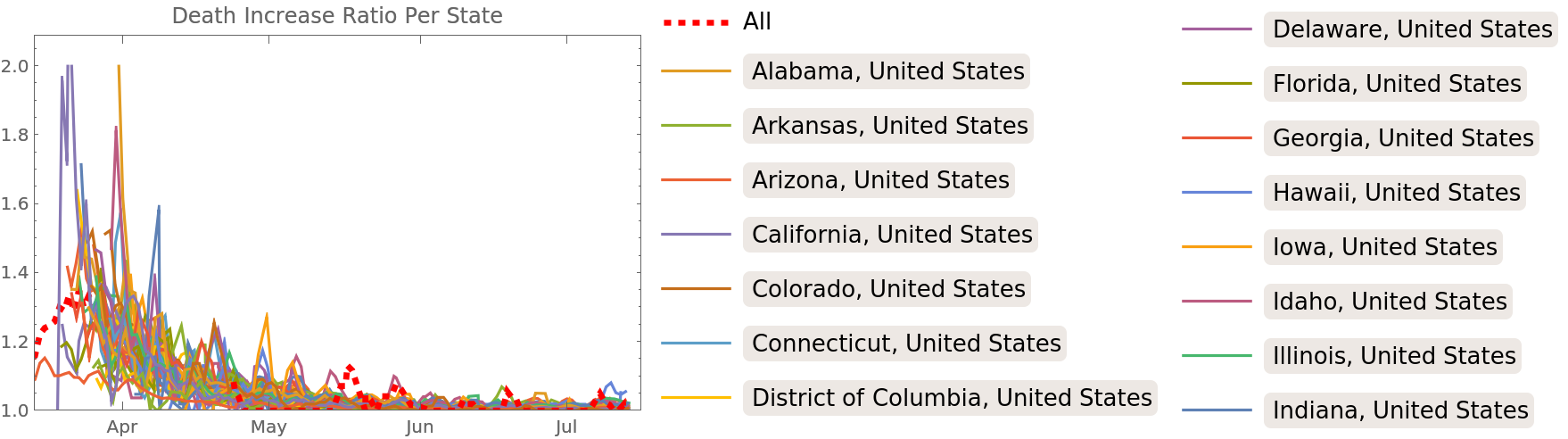#Function Repository Resource:

# COVIDTrackingData

Obsolete. Import data about COVID-19 in the US from the COVID Tracking Project

Contributed by: Bob Sandheinrich  |  Bob
 ResourceFunction["COVIDTrackingData"][type] retrieves data of the specified type from the COVID Tracking Project.

## Details

IMPORTANT NOTE: ResourceFunction["COVIDTrackingData"] ended data collection on March 7th 2021. The API and this resource function will stop working as of May 2021. Consider using the data resource Epidemic Data for Novel Coronavirus COVID-19 or the other resources listed here.
Available data types are "US", "USDaily", "States", "StatesDaily", "StateInformation" and "URLs". The default type is "StatesDaily".
The data is imported from the COVID Tracking Project API.

## Examples

### Basic Examples (6)

Get the latest five days of national data:

 In:=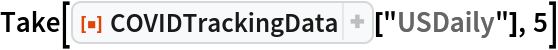Out=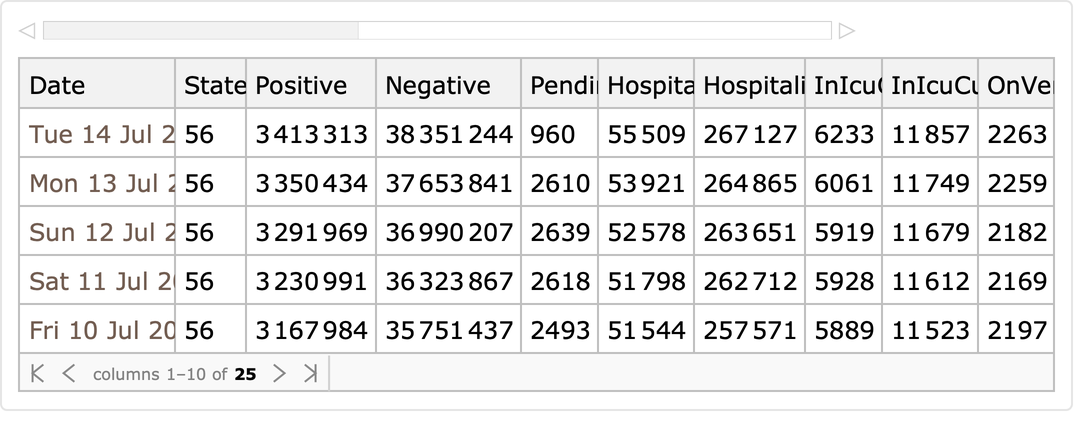Current US data:

 In:=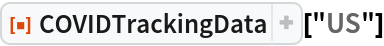Out=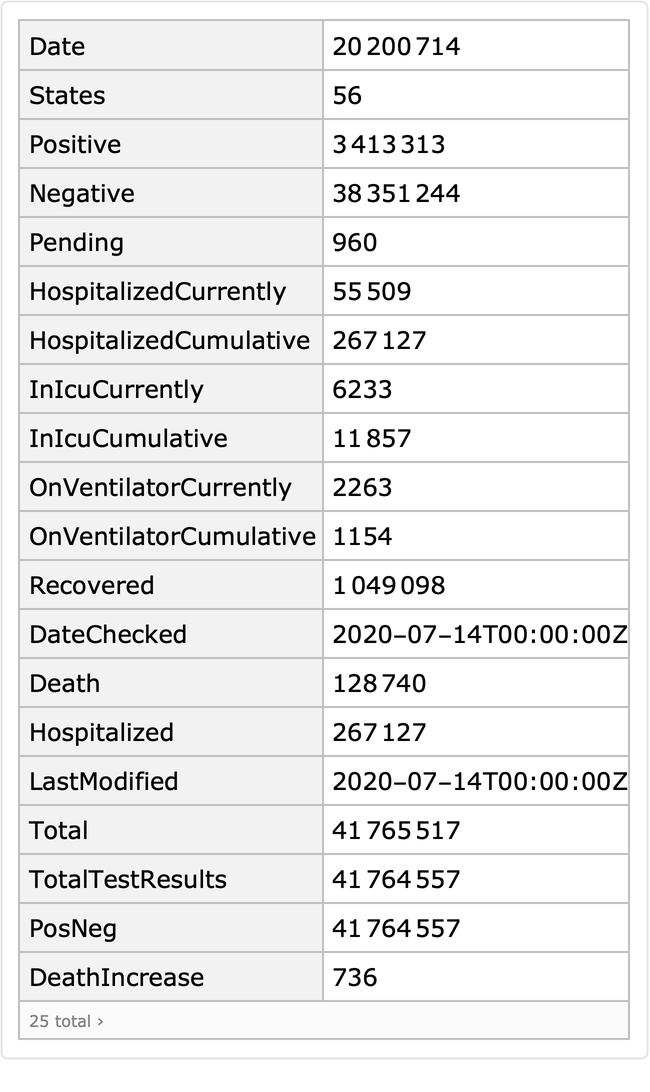Get a sample of daily data on each state:

 In:=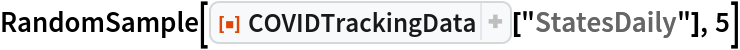Out=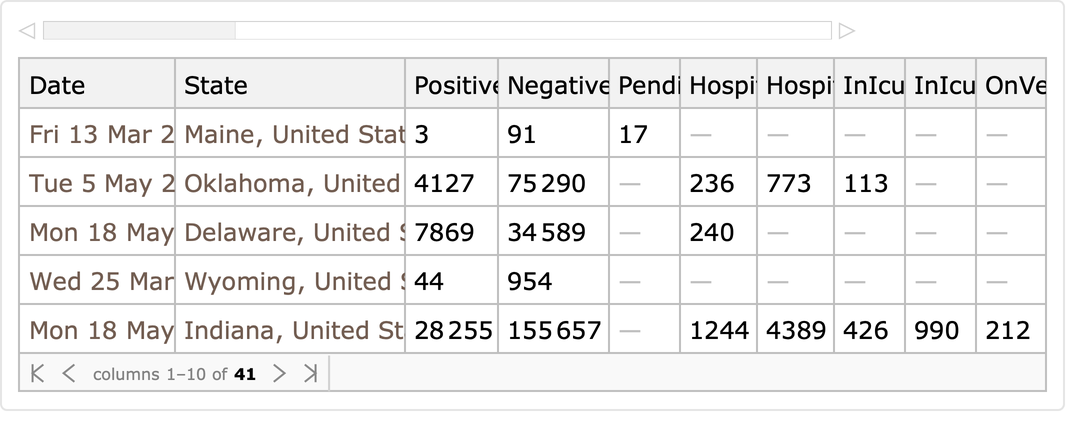Current state data:

 In:=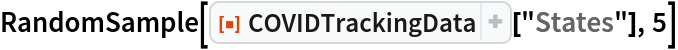Out=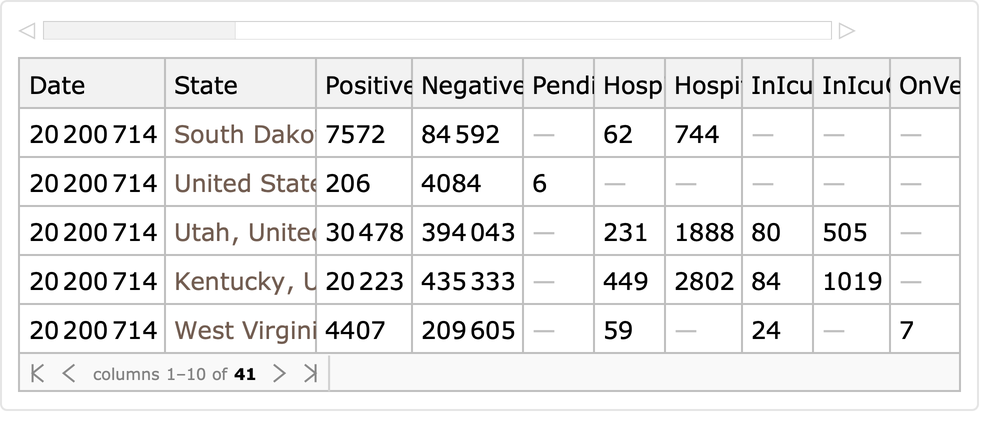Get state information:

 In:=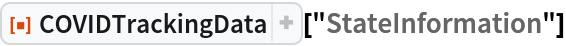Out=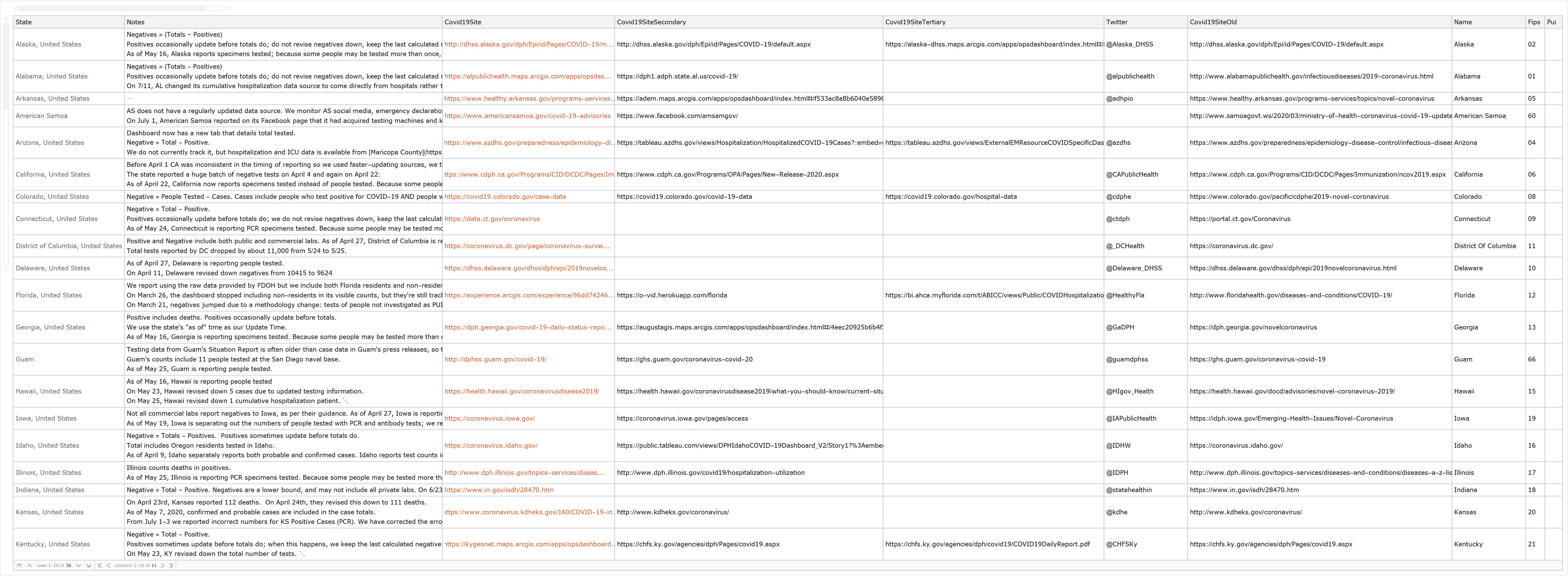Get time series data:

 In:=In:=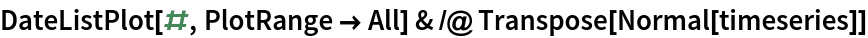Out=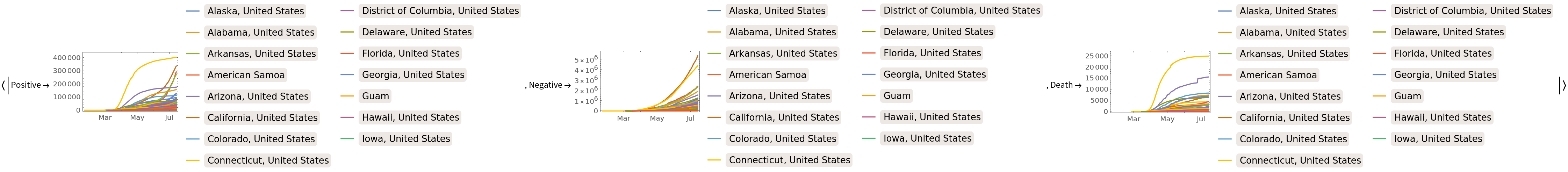### Scope (2)

Plot the number of hospitalizations in the data:

 In:=Out=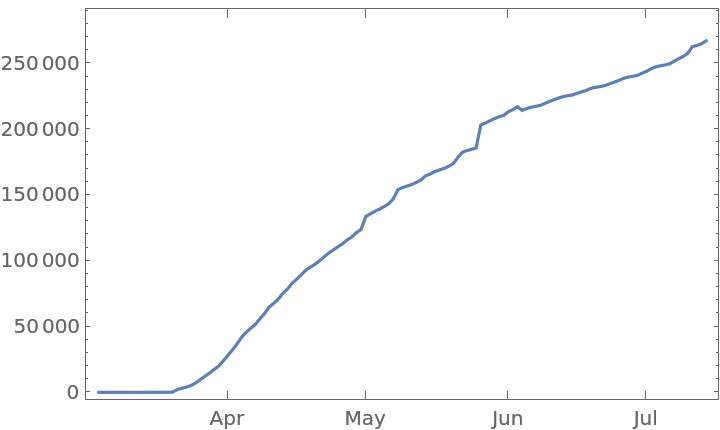Show positive test result counts:

 In:=Out=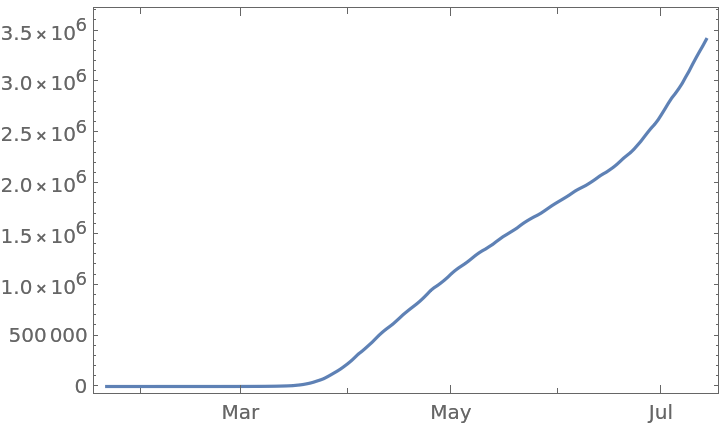### Applications (3)

For each state, find the most recent date with positive and negative testing results:

 In:=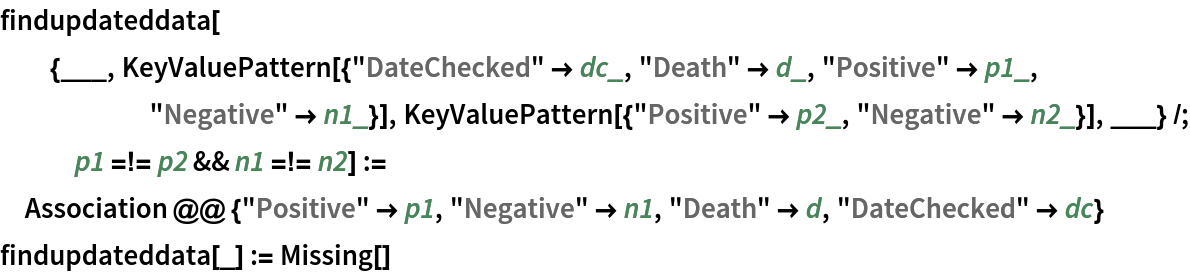In:=Out=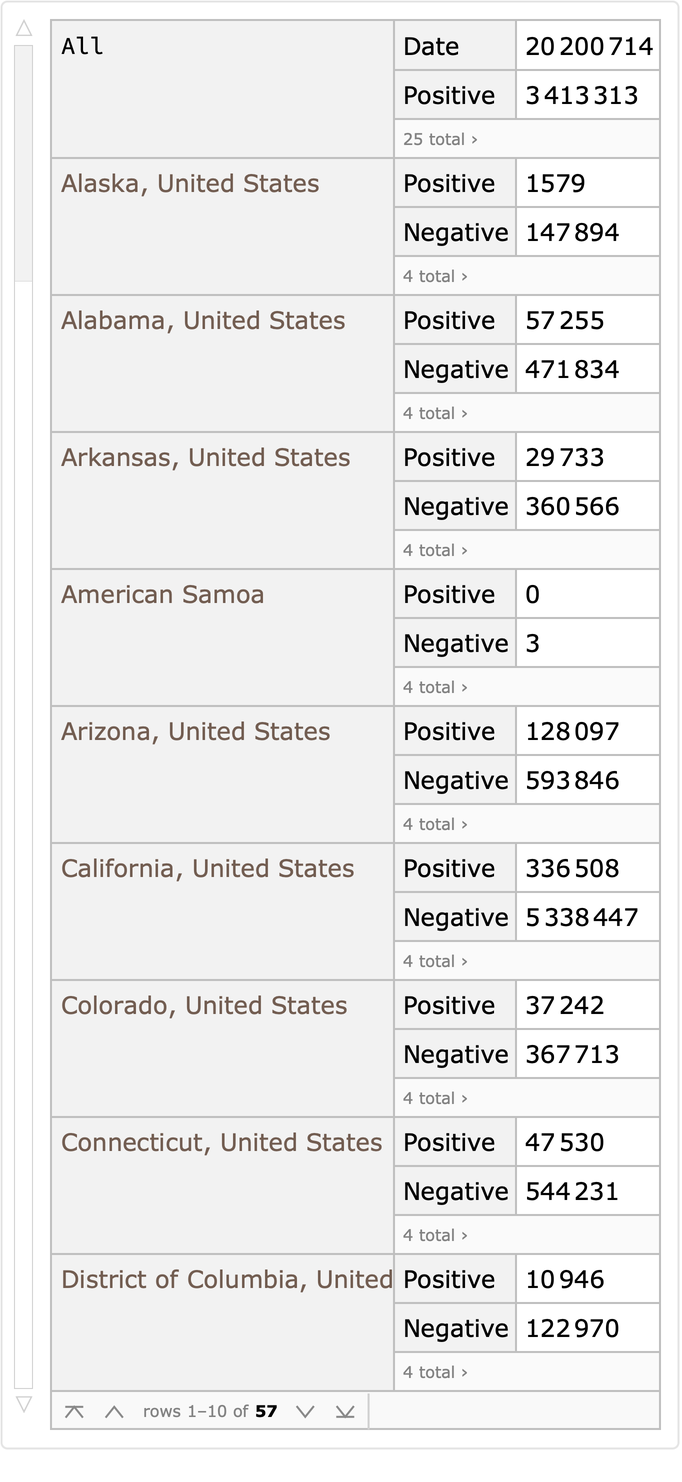See how positive test rates depend on the number of tests:

 In:=Out=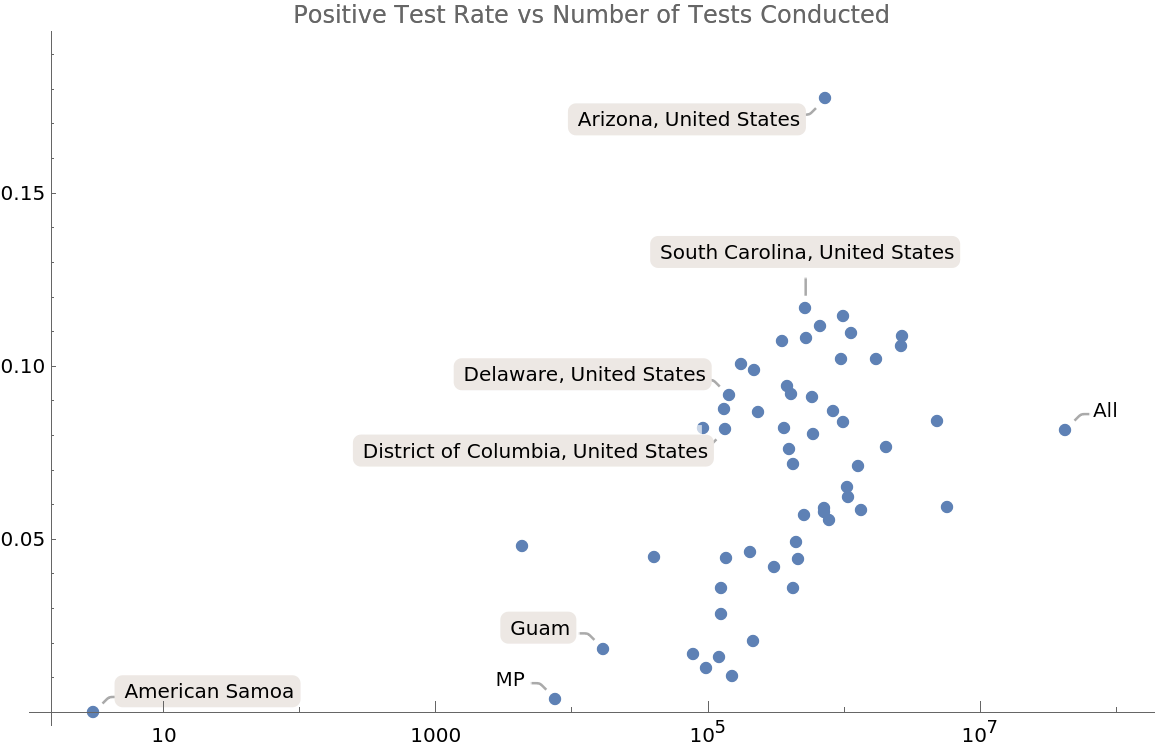See if deaths per positive test are dependent on the amount of testing:

 In:=Out=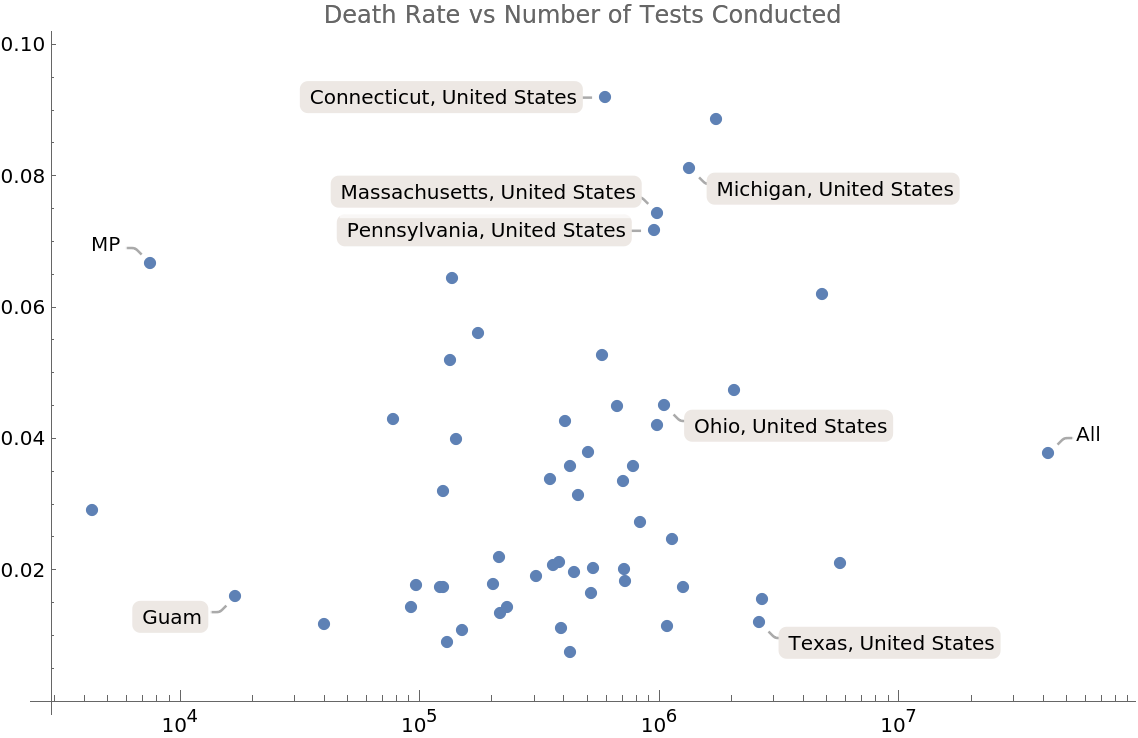See if deaths per positive test are correlated with the positive testing percent:

 In:=Out=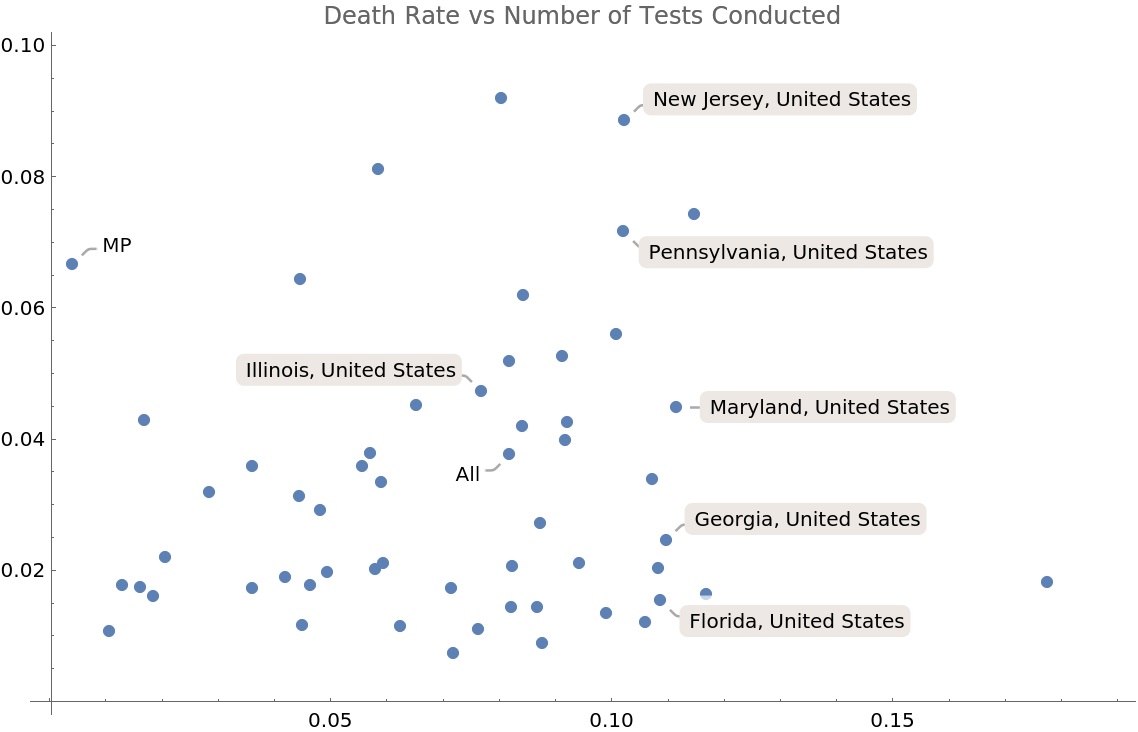Get the new deaths in each state:

 In:=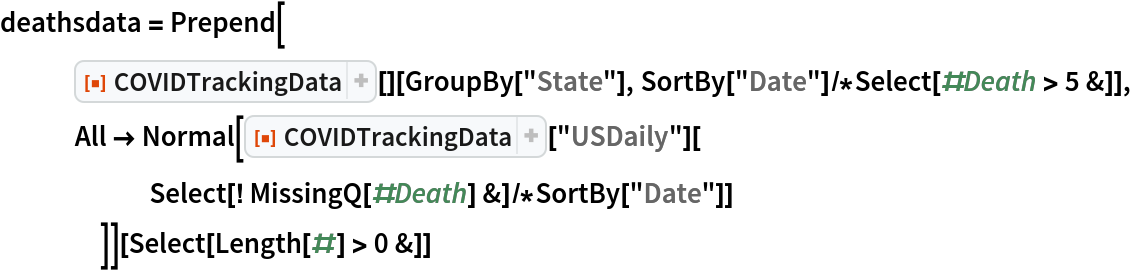Out=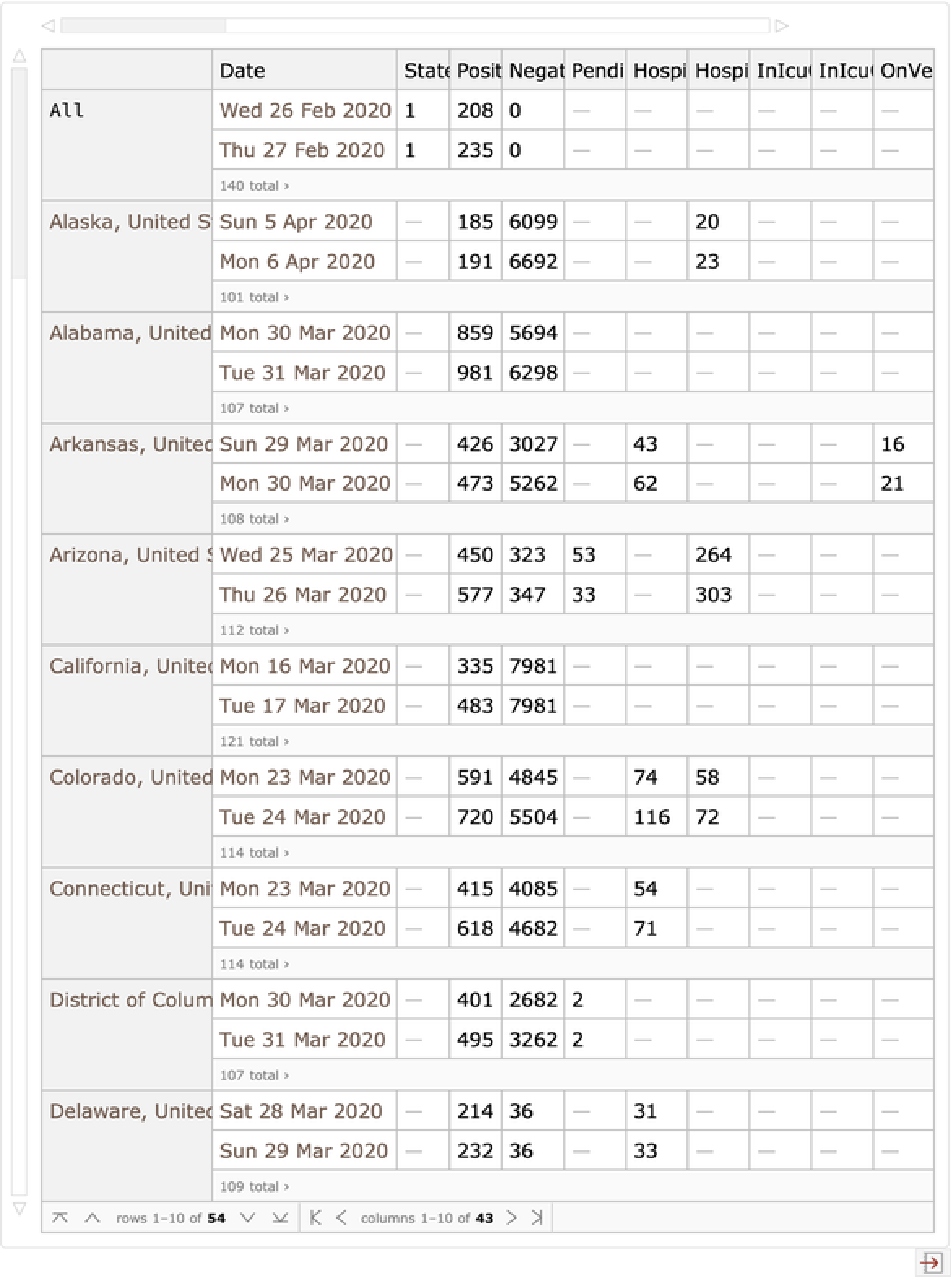Plot the new deaths in each state:

 In:=Out=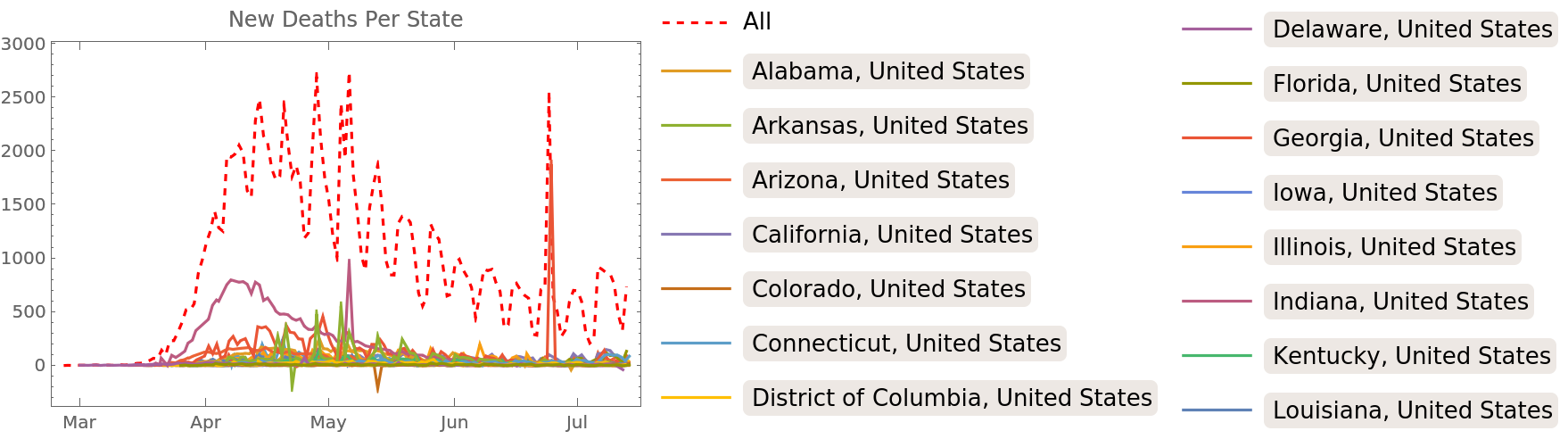Show the smoothed daily proportional increase in deaths in each state:

 In:=Out=